﻿ 上行瑞利衰落信道下SCMA码本设计

# 上行瑞利衰落信道下SCMA码本设计Design of SCMA Codebook for Uplink Rayleigh Fading Channel

Abstract: Sparse code multiple access (SCMA) is a competitive non-orthogonal code domain multiple access technology. By combining modulation with sparse spread spectrum, it can effectively improve the spectral efficiency of communication system. In this paper, a new optimization scheme of codebook design for uplink Rayleigh fading channel is proposed to solve the existing problems in codebook design. Firstly, the parent constellation design is extended from the real number domain to the complex number domain to improve the freedom of codebook design. Secondly, in order to maximize the minimum product distance, the parameters of the codebook were optimized by genetic algorithm. Simulation results show that the BER performance of the proposed codebook is better than that of previous codebooks in uplink Rayleigh fading channel.

1. 引言

SCMA方案由Hosein Nikopour等人在2013年正式提出，文献  系统的提出了一种基于格星座的多阶段次优的SCMA码本设计方法，规范了码本设计步骤，此后大多数学者按照该设计思路对码本设计进行研究。文献  提出了一种基于星形正交振幅度调制(star quadrature amplitude modulation, Star-QAM)的码本设计方案，该方法主要通过增大码字间的最小欧式距离对码本进行优化，该码本在高斯信道下性能得到明显提升，但在瑞利信道中效果不是很理想。文献  提出了上行瑞利衰落信道的SCMA多用户码本设计方案，通过将SCMA系统等效为MIMO系统，以最大化系统截止概率为准则设计码本，生成的码本在瑞利信道下有很好的表现。

2. SCMA系统模型及码本设计理论

2.1. SCMA系统模型

$y=\underset{j=1}{\overset{J}{\sum }}diag\left({h}_{j}\right){x}_{j}+n$ (1)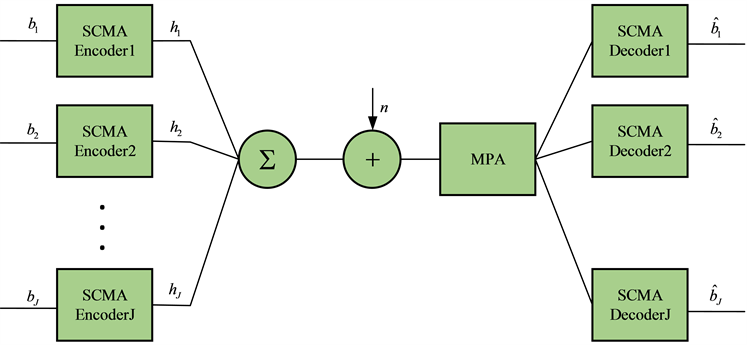Figure 1. SCMA uplink communication system model

2.2. 码本设计理论

$f:{\mathbb{B}}^{{\mathrm{log}}_{2}\left(M\right)}\to \chi ,x=f\left(b\right)$ (2)

$g:{\mathbb{B}}^{{\mathrm{log}}_{2}\left(M\right)}\to \mathcal{C},c=g\left(b\right)$ (3)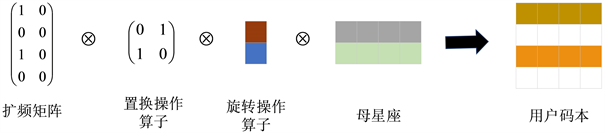Figure 2. Suboptimal SCMA codebook design process

2.3. 最小乘积距离

$p\left({x}^{1}\to {x}^{2}|h\right)=Q\left(\sqrt{\frac{{|h\left({x}^{1}-{x}^{2}\right)|}^{2}}{2{N}_{0}}}\right)$ (4)

$p\left(x\left(1\right)|h\right)=\underset{c=1}{\overset{{M}^{J}-1}{\sum }}Q\left(\sqrt{\frac{{|h\left(x\left(1\right)-x\left(c\right)\right)|}^{2}}{2{N}_{0}}}\right)$ (5)

$p\left(x\to y\right)\le \frac{1}{2}\underset{k=1}{\overset{K}{\prod }}\frac{1}{1+\frac{{\left({x}_{k}-{y}_{k}\right)}^{2}}{8{N}_{0}}}$ (6)

$p\left(x\to y\right)\le \frac{1}{2}\frac{1}{{\left(\frac{\eta }{8}\frac{{E}_{b}}{{N}_{0}}\right)}^{l}{d}_{p}^{\left(l\right)}{\left(x,y\right)}^{2}}$ (7)

$\text{MPD}=\underset{j}{\mathrm{min}}\left\{\underset{k=1,{x}_{j,p}^{k}\ne {x}_{j,q}^{k}}{\overset{K}{\prod }}|{x}_{j,p}^{k}-{x}_{j,q}^{k}|,1\le p (8)

3. 瑞利衰落信道下SCMA码本设计及优化

3.1. SCMA码本设计

${C}_{2×4}=\left(\begin{array}{cccc}\rho \omega {\text{e}}^{j\theta }& \omega & -\omega & -\rho \omega {\text{e}}^{j\theta }\\ -\omega & \rho \omega {\text{e}}^{j\theta }& -\rho \omega {\text{e}}^{j\theta }& \omega \end{array}\right)$ (9)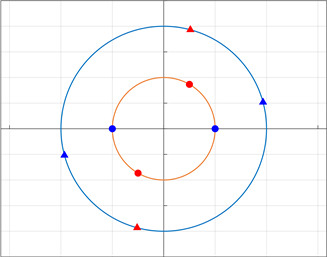Figure 3. Parental constellation structure

${\chi }_{j}=ezc\left(\text{diag}\left({S}_{j}\right)\right)\mathcal{C}$ (10)

$\left(\begin{array}{cccc}{a}_{1}& 0& 0& 0\\ 0& {a}_{2}& 0& 0\\ 0& 0& 0& 0\\ 0& 0& 0& 0\end{array}\right)\to \left(\begin{array}{cc}{a}_{1}& 0\\ 0& {a}_{2}\\ 0& 0\\ 0& 0\end{array}\right)$ (11)

${S}_{4×6}=\left[\begin{array}{cccccc}0& {z}_{1}& {z}_{2}& 0& {z}_{3}& 0\\ {z}_{1}& 0& {z}_{2}& 0& 0& {z}_{3}\\ 0& {z}_{3}& 0& {z}_{2}& 0& {z}_{1}\\ {z}_{3}& 0& 0& {z}_{2}& {z}_{1}& 0\end{array}\right].$ (12)

3.2. 瑞利衰落信道下码本优化

$\begin{array}{ll}\underset{\phi ,\rho ,\theta ,\omega }{\mathrm{max}}\text{ }\hfill & \text{MPD}\hfill \\ \text{s}\text{.t}\text{.}\hfill & 0<{\phi }_{i}<\pi ,\text{ }\forall i=1,2,\cdots ,{d}_{f}\hfill \\ \hfill & 0<\theta <\pi \hfill \\ \hfill & \rho \ge 1,\hfill \end{array}$ (13)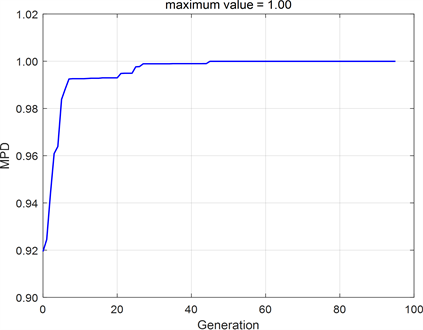Figure 4. Optimization results of genetic algorithm

4. 仿真Table 1. Summative comparison of different codebooksTable 2. SCMA codebook simulation parameters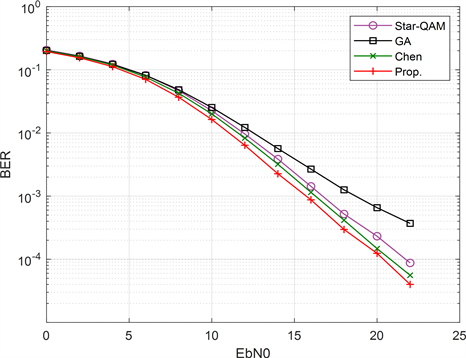Figure 5. Comparison of BER performance between different codebooks in uplink Rayleigh fading channel

5. 结论

 Ding, Z,, Lei, X., Karagiannidis, G.K., et al. (2017) A Survey on Non-Orthogonal Multiple Access for 5G Networks: Research Challenges and Future Trends. IEEE Journal on Selected Areas in Communications, 35, 2181-2195.
https://doi.org/10.1109/JSAC.2017.2725519

 Nikopour, H. and Baligh, H. (2013) Sparse Code Multiple Access. 2013 IEEE 23th Annual International Symposium on Personal, Indoor, and Mobile Radio Communications (PIMRC), 8-11 September 2013, London.
https://doi.org/10.1109/PIMRC.2013.6666156

 Taherzadeh, M., Nikopour, H., Bayesteh, A., et al. (2013) SCMA Codebook Design. 2013 IEEE 80th Vehicular Technology Conference (VTC2013-Fall), 14-17 September 2014, Vancouver.
https://doi.org/10.1109/VTCFall.2014.6966170

 Yu, L., Lei, X., Fan, P., et al. (2015) An Optimized Design of SCMA Codebook Based on Star-QAM Signaling Constellations. 2015 International Conference on Wireless Communications & Signal Processing (WCSP), 15-17 October 2015, Nanjing.
https://doi.org/10.1109/WCSP.2015.7341311

 Bao, J., Ma, Z., Ding, Z., et al. (2016) On the Design of Multiuser Codebooks for Uplink SCMA Systems. IEEE Communications Letters, 20, 1920-1923.
https://doi.org/10.1109/LCOMM.2016.2596759

 Alam, M. and Zhang, Q. (2017) Performance Study of SCMA Codebook Design. 2017 IEEE Wireless Communications and Networking Conference (WCNC), 19-22 March 2017, San Francisco.
https://doi.org/10.1109/WCNC.2017.7925767

 Van De Beek, J. and Popovic, B.M. (2009) Multiple Access with Low-Density Signatures. GLOBECOM 2009-2009 IEEE Global Telecommunications Conference, 30 November-4 December 2009, Honolulu.
https://doi.org/10.1109/GLOCOM.2009.5425243

 Boutros, J., Viterbo, E., Rastello, C., et al. (1996) Good Lattice Constellations for Both Rayleigh Fading and Gaussian Channels. IEEE Transactions on Information Theory, 42, 502-518.
https://doi.org/10.1109/18.485720

 Hoshyar, R., Wathan, F.P. and Tafazolli, R. (2008) Novel Low-Density Signature for Synchronous CDMA Systems over AWGN Channel. IEEE Transactions on Signal Processing, 56, 1616-1626.
https://doi.org/10.1109/TSP.2007.909320

 Klimentyev, V.P. and Sergienko, A.B. (2017) SCMA Codebooks Optimization Based on Genetic Algorithm. European Wireless 2017; 23th European Wireless Conference, 17 August 2017.

 Chen, Y. and Chen, J. (2020) On the Design of Near-Optimal Sparse Code Multiple Access Codebooks. IEEE Transactions on Communications, 68, 2950-2962.
https://doi.org/10.1109/TCOMM.2020.2974213

Top